A particle is incident with a constant energy...
A particle is incident with a constant energy E on a one dimensional potential barrier as shown in the figure. The wave function in regions I and II are respectively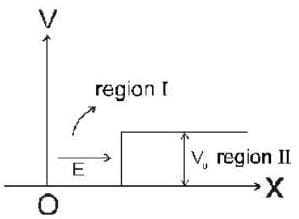• a)
decaying, oscillatory
• b)
Oscillatory, oscillatory
• c)
oscillatory, decaying
• d)
decaying, decaying
A particle is incident with a constant energy E on a one dimensional p...
Schrodinger equation in region I is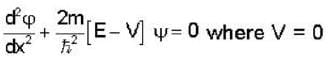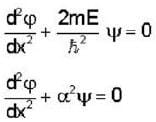The wave function in region I is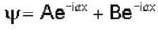is oscillatory
Schrodinger equation in region II is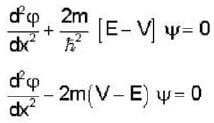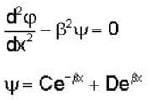When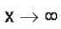then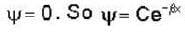and this wavefunction in region II is decaying.
 1 Crore+ students have signed up on EduRev. Have you?
1 Crore+ students have signed up on EduRev. Have you?

### Learn this topic in detailGATE Physics Mock Test Series - 4 60 Ques | 180 MinsView courses related to this question

### Quick links for GATE exam850+
Video Lectures2500+
Revision Notes600+
Online Tests34+
Courses
A particle is incident with a constant energy E on a one dimensional potential barrier as shown in the figure. The wave function in regions I and II are respectivelya)decaying, oscillatoryb)Oscillatory, oscillatoryc)oscillatory, decayingd)decaying, decayingCorrect answer is option 'C'. Can you explain this answer?
Question Description
A particle is incident with a constant energy E on a one dimensional potential barrier as shown in the figure. The wave function in regions I and II are respectivelya)decaying, oscillatoryb)Oscillatory, oscillatoryc)oscillatory, decayingd)decaying, decayingCorrect answer is option 'C'. Can you explain this answer? for GATE 2023 is part of GATE preparation. The Question and answers have been prepared according to the GATE exam syllabus. Information about A particle is incident with a constant energy E on a one dimensional potential barrier as shown in the figure. The wave function in regions I and II are respectivelya)decaying, oscillatoryb)Oscillatory, oscillatoryc)oscillatory, decayingd)decaying, decayingCorrect answer is option 'C'. Can you explain this answer? covers all topics & solutions for GATE 2023 Exam. Find important definitions, questions, meanings, examples, exercises and tests below for A particle is incident with a constant energy E on a one dimensional potential barrier as shown in the figure. The wave function in regions I and II are respectivelya)decaying, oscillatoryb)Oscillatory, oscillatoryc)oscillatory, decayingd)decaying, decayingCorrect answer is option 'C'. Can you explain this answer?.
Solutions for A particle is incident with a constant energy E on a one dimensional potential barrier as shown in the figure. The wave function in regions I and II are respectivelya)decaying, oscillatoryb)Oscillatory, oscillatoryc)oscillatory, decayingd)decaying, decayingCorrect answer is option 'C'. Can you explain this answer? in English & in Hindi are available as part of our courses for GATE. Download more important topics, notes, lectures and mock test series for GATE Exam by signing up for free.
Here you can find the meaning of A particle is incident with a constant energy E on a one dimensional potential barrier as shown in the figure. The wave function in regions I and II are respectivelya)decaying, oscillatoryb)Oscillatory, oscillatoryc)oscillatory, decayingd)decaying, decayingCorrect answer is option 'C'. Can you explain this answer? defined & explained in the simplest way possible. Besides giving the explanation of A particle is incident with a constant energy E on a one dimensional potential barrier as shown in the figure. The wave function in regions I and II are respectivelya)decaying, oscillatoryb)Oscillatory, oscillatoryc)oscillatory, decayingd)decaying, decayingCorrect answer is option 'C'. Can you explain this answer?, a detailed solution for A particle is incident with a constant energy E on a one dimensional potential barrier as shown in the figure. The wave function in regions I and II are respectivelya)decaying, oscillatoryb)Oscillatory, oscillatoryc)oscillatory, decayingd)decaying, decayingCorrect answer is option 'C'. Can you explain this answer? has been provided alongside types of A particle is incident with a constant energy E on a one dimensional potential barrier as shown in the figure. The wave function in regions I and II are respectivelya)decaying, oscillatoryb)Oscillatory, oscillatoryc)oscillatory, decayingd)decaying, decayingCorrect answer is option 'C'. Can you explain this answer? theory, EduRev gives you an ample number of questions to practice A particle is incident with a constant energy E on a one dimensional potential barrier as shown in the figure. The wave function in regions I and II are respectivelya)decaying, oscillatoryb)Oscillatory, oscillatoryc)oscillatory, decayingd)decaying, decayingCorrect answer is option 'C'. Can you explain this answer? tests, examples and also practice GATE tests.(Scan QR code)